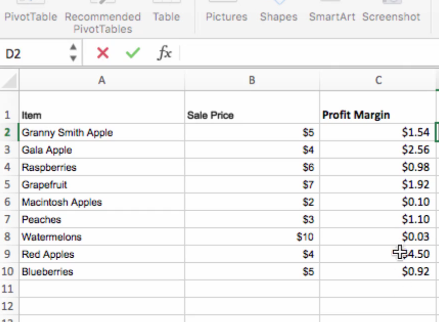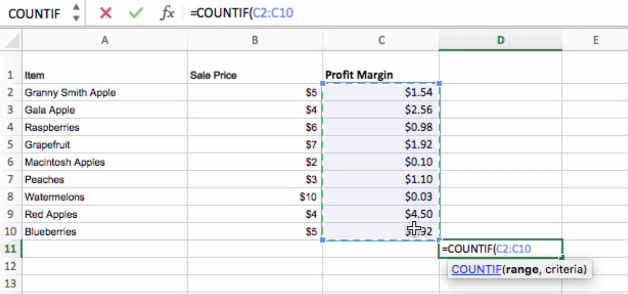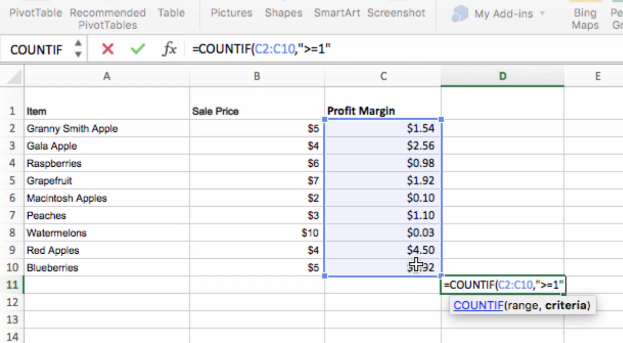## Popular Functions in Excel: Lesson 3 – “COUNTIF"## In part three of our miniseries on the most popular Excel functions, we’ll cover the “COUNTIF” function, which counts the number of cells that meet a certain criteria.

If you missed it, check out part one (“IF”) and part two (“SUM”) of our series.

Using the COUNTIF function, you can gather a tally of certain types of cells using a logical IF condition as part of the function.

Let’s look at a basic example of how you might use the COUNTIF function. Let’s imagine that you want to know how many items in this spreadsheet below have a profit margin greater than \$1. That’s easy enough using the COUNTIF function.• So, we start by just typing =COUNTIF( and then we’re going to have to enter two specific terms in order to use the function. The first is the range of cells that you want to include in the analysis. This is easy enough, because like any other function in Excel you can either manually select the range once you’ve opened the function, or you can simply type it in.• Then, you’ll put a comma after your range and then specify the condition that has to be met in order for a cell to be counted. So if a cell meets whatever criteria we specify here, it will count as 1 cell in the overall count. If it does not, it will be ignored. Since we want to find all the profit margins that are greater or equal to 1, we’ll put “>=1” in this term and close the parentheses.• We now have a count of the number of cells with a profit margin greater than one.• Keep in mind that you can use almost any type of criteria for specifying what type of cells you want to count. For instance, we can also specify a string in that criteria, such as “Granny Smith Apple” and then we can see how many times Granny Smith Apple appears in our list.